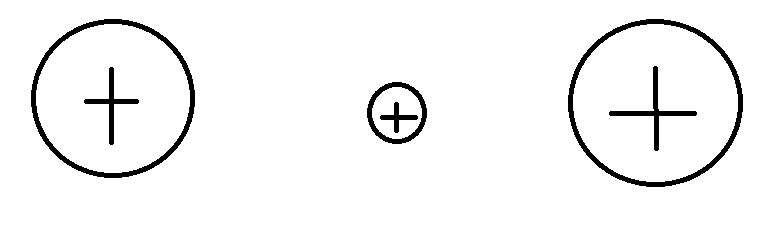# Confusion regarding Electric Potential Energy and Work

Abu
Hi everyone.

I've been doing a lot of reading regarding electric potential and electric potential energy. Unfortunately, I have a lot of confusion regarding this topic, as I keep receiving different information. My main confusion is regarding the signs, positive or negative, of work and it's respective potential energy... as well as what is doing the work in the first place.
I have seen some people write the formulas of electric potential energy and work as:

W=-PE = -qΔV
And I've also seen people write it as:
W=PE = qΔV

To clarify my confusion, let's say that we have two positive charges that are fixed across from each other, with another smaller positive charge that is in between them, like this:And we move the smaller positive charge to the right
If we go by the logic of the first formula, which states:
W=-PE = -qΔV
Then
1. The change in PE will be positive, because it is going from an area of lower potential to one of higher (closer to the large positive charge on the right)
2. The value for q will be positive because the small charge is a positive charge
3. The work done will be negative, according to the formula
4. The PE will also be positive because you will have -PE = -qΔV and the negative signs cancel each other out
Now my confusion is why is the work done negative? I am guessing, and correct me if I am wrong, that when the charge is moved closer to the right, the value for the electric field becomes larger to the point that overall the electric field is pointing to the left. As a result, since the charge's displacement is to the right and the overall electric field vectors point to the left, then the work done is negative. However, I'm not trying to turn this into a homework thread, but a similar problem showed that the answer for the work done was positive... which conflicts with my previous logic and what the formula above states.

If we use the second variation of the formula, which states:
W=PE = qΔV
Then
1. The change in PE will be positive
2. The value for q is still positive
3. The work done will be positive
I don't know which version of the formula is correct, or perhaps I should say I don't know what is doing the work on the small charge.

I know this is kind of a lengthy post, but it has been on my mind for a while now. I appreciate all feedback I receive on this. Thank you very much everyone. I can also add more diagrams if my question isn't clear.

Last edited: